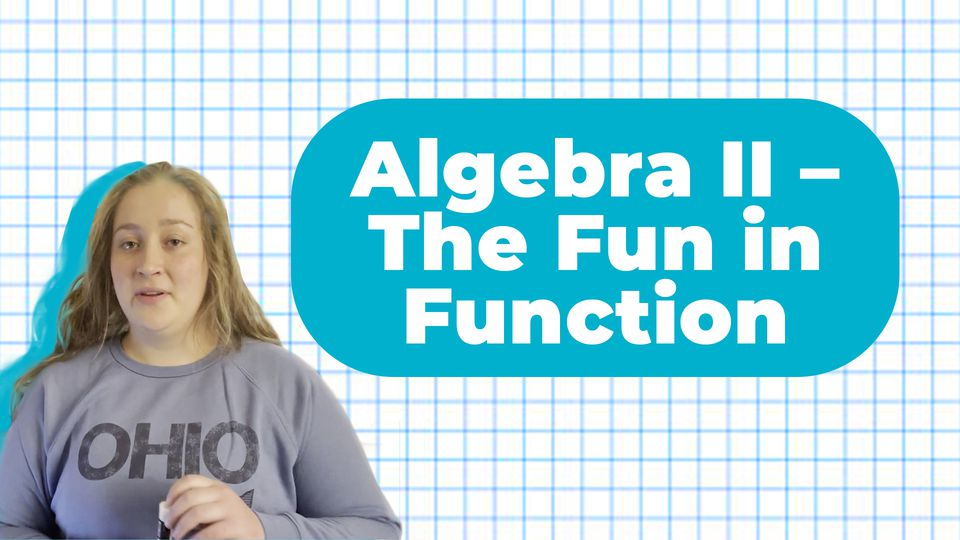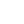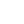Preview the Course
1h 14m

# Algebra 2 –The Fundamental Theorem of Algebra

by Elizabeth C. | Math FREE8 Lessons 1:14:46
1.1. Introduction 12:00
2.2. Review of Synthetic Substitution, Factoring Polynomials and Leading Coefficients 8:26
3.3. Synthetic Division 4:14
4.4. Explanation of Finding Rational Zeros with Several Practice Problems 14:36
5.5. Overview of the Fundamental Theorem 3:43
6.6. Practice Problems applying the Fundamental Theorem 7:52
7.7. Overview of the Conjugate Theorem and Several Practice Problems to Apply It 11:23
8.8. Overview Descartes's Rule of Signs, Explanation of When It Is Important, and A Practice Problem to Apply the Theorem 12:32

1h 14m

14

Students

NGSS Standards

FREE

Course

The fundamental theorem of algebra is the foundation for higher level math. This class will explore multi-variable functions and the basics of trigonometry; this translates to understanding rates, building foundational knowledge for STEM courses (in proportions, data interpretation, etc), developing critical thinking skills, and covering a portion of the math questions students will see on the SAT/ACT.

Tags:

• Algebra II
• Functions
• Graphing
• Highschool
• Interpreting Data
• Math
• Polynomials
• Sine Functions
• Test Prep
• Trigonometry

## Instructor

Hello! I'm Beth and I recently graduated from Northeastern University in Boston with a B.S. in Chemical Engineering. I currently work as the materials and chemical engineer at a startup in the Boston area that works on remediating industrial mining waste. I have been tutoring for nearly 8 years, starting back in Columbus, OH where I helped at the Metropolitan library, and spanning into college at the Connections Lab at Northeastern University. My favorite part of tutoring is helping students reach their full potential and get them to those "AH HA" moments.

See full profile